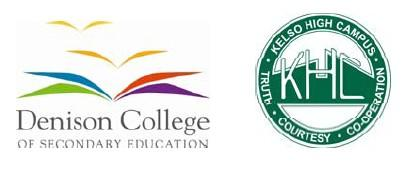# 8sw2 Yearly Science Exam

37 Questions | Total Attempts: 55SettingsCreate your own QuizYear 8 - 2016 End of Year Science Exam

• 1.
The particle of an atom with a positive charge is known as the:
• A.

Neutron

• B.

Proton

• C.

Electron

• D.

Particle

• 2.
The particles that move around the nucleus of an atom are called:
• A.

Neutrons

• B.

Protons

• C.

Electrons

• D.

Ions

• 3.
All atoms are made up of what 3 basic components?
• A.

Nucleus, protons and electrons

• B.

Ions, protons and electrons

• C.

Electrons, protons and neutrons

• D.

Elements, compounds and mixtures

• 4.
In the table shown which letter best describes the characteristics of a metal?
• A.

A

• B.

B

• C.

C

• 5.
In the table shown which letter best describes the characteristics of a non-metal?
• A.

A

• B.

B

• C.

C

• 6.
In the picture shown, what is represented by line A?
• A.

Atomic number

• B.

Atomic mass

• C.

Number of protons

• D.

The group number

• 7.
In the picture shown, which line represents the symbol for the element?
• A.

B

• B.

A

• C.

C

• D.

D

• 8.
An element be broken down into a simpler substance
• A.

True

• B.

False

• 9.
In the picture shown, what does the box labelled A represent?
• A.

Pure Element –only one typeof atom present

• B.

Mixture of two elements –two types of uncombined atoms present.

• C.

Pure compound –only one type of compound present

• D.

Mixture of two compounds –two types of compounds present

• E.

Mixture of a compound and an element.

• 10.
In the picture shown, what does the box labelled B represent?
• A.

Pure Element –only one typeof atom present

• B.

Mixture of two elements –two types of uncombined atoms present.

• C.

Pure compound –only one type of compound present

• D.

Mixture of two compounds –two types of compounds present

• E.

Mixture of a compound and an element.

• 11.
In the picture shown, what does the box labelled C represent?
• A.

Pure Element –only one typeof atom present

• B.

Mixture of two elements –two types of uncombined atoms present.

• C.

Pure compound –only one type of compound present

• D.

Mixture of two compounds –two types of compounds present

• E.

Mixture of a compound and an element.

• 12.
The chemical formula for the compound salt is NaCl. Salt contains the elements:(hint = use the picture shown)
• A.

Sodium and carbon

• B.

Sodium and chlorine

• C.

Chlorine and nitrogen

• D.

Nitrogen and carbon

• 13.
Most of the worlds electricity comes from the burning of:
• A.

Alcohol

• B.

Coal

• C.

Oil

• D.

Natural gas

• 14.
Coal stores energy. The energy originally came from:
• A.

The Earth

• B.

The sun

• C.

Chemicals

• D.

Rocks

• 15.
A natural fuel formed in the geological past from the remains of living organisms is known as:
• A.

A fossil fuel

• B.

Solar energy

• C.

Geothermal

• D.

Biomass

• 16.
Which of these resources is non-renewable?
• A.

Natural gas

• B.

Wind

• C.

Solar energy

• D.

Hydroelectricity

• 17.
Plants obtain the energy to make food from:
• A.

Water

• B.

Air

• C.

Soil

• D.

The sun

• 18.
Which of the following is a fossil fuel?
• A.

Oil

• B.

Iron ore

• C.

Solar energy

• D.

Hydrogen

• 19.
Which of the following is NOT a chemical change?
• A.

Burning a match

• B.

Dissolving sugar in tea

• C.

Ripening of a banana

• D.

Cooking a cake

• 20.
Potential Energy (PE) is:
• A.

The energy caused by the movement of an object

• B.

Energy that is stored (like compressed springs)

• C.

The energy that is used when a light is switched on

• D.

The energy released when a runner gets hot

• 21.
Batteries are stores of:
• A.

Electrical energy

• B.

Chemical energy

• C.

Kinetic energy

• D.

Acidic energy

• 22.
Food is a source of:
• A.

Electrical energy

• B.

Chemical energy

• C.

Kinetic energy

• D.

Thermal energy

• 23.
This image shows which method of heat transfer:
• A.

Conduction

• B.

Convection

• C.

• D.

Thermal energy

• 24.
This image shows which method of heat transfer:
• A.

Conduction

• B.

Convection

• C.

• D.

Thermal energy

• 25.
In which situation below is heat being transferred mainly by radiation?
• A.

From a hot clothes iron to your hand held close to it

• B.

From hot plate on the stove to the soup in the saucepan

• C.

From a hot car door to your hand placed on the metal

• D.

From a hot drink to the end of a metal spoon used to stir it

Related TopicsBack to top
×

Wait!
Here's an interesting quiz for you.